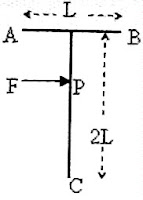## Monday, September 10, 2007

### Solution to Multiple Choice Questions on Centre of Mass

Two multiple choice questions on centre of mass were given to you for practice yesterday. Here is the solution along with the questions:

(1) A boy weighing 40 kg is standing on a wooden log of mass 500 kg floating on still water in a lake. The distance of the boy from the shore is 12 m. The viscous force exerted by water on the wooden log may be neglected. If the boy walks slowly along the wooden log through 2 m towards the shore, the centre of mass of the system (wooden log and the boy) will move with respect to the shore through a distance

(a) 2 m (b) 1.25 m (c) 0.25 m (d) 0.16 m (e) zero

You should have worked this out within seconds. The centre of mass will be unaffected with respect to external fixed points if there are no external forces acting on the system. So, the correct option is (e).

(2) A T-shaped object with dimensions shown in the figure, is lying on a smooth floor. A force F is applied at the point P parallel to AB, such that the object has only translational motion without rotation. Find the location of P with respect to C

(a) L (b) 4L/3 (c) 3L/2 (d) 2L/3The point P must be the centre of mass of the T-shaped object since the force F does not produce any rotational motion of the object. So, we have to find the distance of the centre of mass from the point C.

The horizontal part of the T-shaped object has length L. If the mass of the horizontal portion is ‘m’, the mass of the vertical portion of the T- shaped object is 2m since its length is 2L. For finding the centre of mass of the T shaped object, it is enough to consider two point masses m and 2m located respectively at the mid points of the horizontal and vertical portions of the T.

Therefore, the T-shaped object reduces to two point masses m and 2m at distances 2L and L respectively from the point C. The distance ‘r’ of the centre of mass of the system from the point C is given by

r = (m1r1 + m2r2)/(m1 + m2) = (m×2L + 2m×L)/(m + 2m) = 4L/3

[ Note that we have used the equation, r = (m1r1 + m2r2)/(m1 + m2) for the position vector r of the centre of mass in terms of the position vectors r1 and r2 of the point masses m1 and m2. We could use the simple equation involving the distances from C since the points are collinear].

1.2.